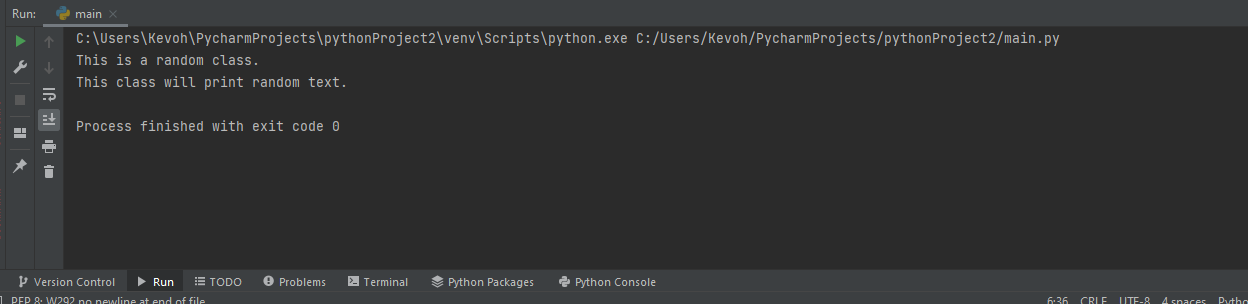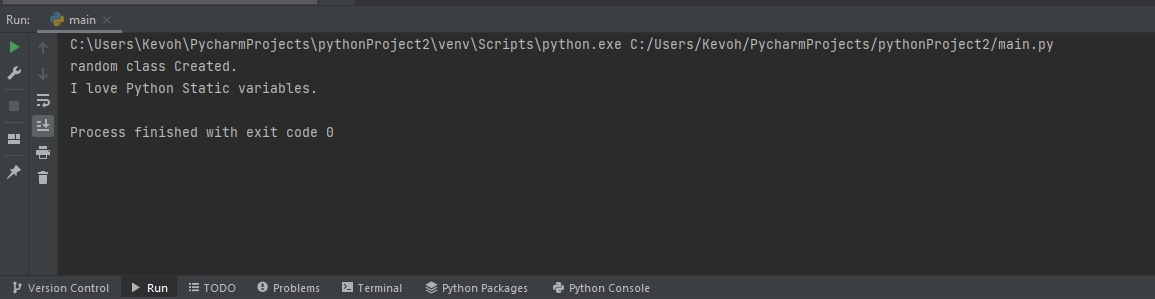# Explain python static variables

Published July 26, 2022

In Python, a static variable is one that is declared inside a specified class but not within a function. This variable can be accessed only via the class in which it is declared. A static variable is often referred to as a class variable. Because these variables are class-specific, they cannot affect the state of an object.

• How to create static variables using the staticmethod()

• How to create static variables using the @staticmethod

## How to create static variables using the staticmethod()

In Python, staticmethod() returns a static variable for a given function. However, these variables are now considered absolute, so using this method is no longer recommended.

To create variables, we first create a class called StaticVar. Using the staticmethod() function, we declare a variable called random as a static variable outside the class. By using the StaticVar class, we can directly call random().

## Example

 class StaticVar:     def random(a):         print(a)         print("My static variable.") StaticVar.random = staticmethod(StaticVar.random) StaticVar.random("a Random class.")

## Output## How to create static variables using the @staticmethod

A static variable can be defined using @staticmethod, which is the most commonly used and modern method. In Python, @staticmethod is a built-in decorator. In Python, a decorator adds new functionality to an existing object without changing its structure. Python uses the @staticmethod decorator to define a static variable inside a class.

## Example

 class StaticVar:     @staticmethod     def random(a):         print(a)         print("I love Python Static variables.") StaticVar.random("random class Created.")

## OutputArticle Contributed By :192 Views
Projects By category
Categories